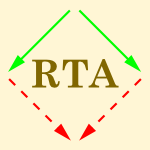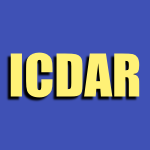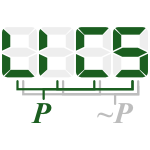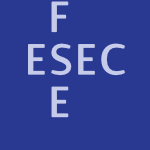`Used together with:`
space (10)
time (5)
sort (4)
problem (4)
use (4)

## Stem logarithm\$ (all stems)

### 36 papers:ICALP-v1-2015-CohenS
Zero-Fixing Extractors for Sub-Logarithmic Entropy (GC, IS), pp. 343–354.CSL-2015-Mazza
Simple Parsimonious Types and Logarithmic Space (DM), pp. 24–40.DRR-2014-NagyL #distance #similarity
Form similarity via Levenshtein distance between ortho-filtered logarithmic ruling-gap ratios (GN, DPL), pp. 902106–8.RTA-TLCA-2014-AubertB #unification
Unification and Logarithmic Space (CA, MB), pp. 77–92.DATE-2013-RustLP #architecture #complexity #using
Low complexity QR-decomposition architecture using the logarithmic number system (JR, FL, SP), pp. 97–102.PODS-2013-ChenM #classification #complexity #query
The fine classification of conjunctive queries and parameterized logarithmic space complexity (HC, MM), pp. 309–320.LATA-2012-Axelsen #automaton #finite #multi
Reversible Multi-head Finite Automata Characterize Reversible Logarithmic Space (HBA), pp. 95–105.ICDAR-2011-HasegawaT #correlation
A Shape Descriptor Combining Logarithmic-Scale Histogram of Radon Transform and Phase-Only Correlation Function (MH, ST), pp. 182–186.CSL-2011-GroheGHL #logic #recursion
L-Recursion and a new Logic for Logarithmic Space (MG, BG, AH, BL), pp. 277–291.DLT-2010-GeffertP #automaton
Two-Way Unary Automata versus Logarithmic Space (VG, GP), pp. 197–208.STOC-2007-PeikertR #worst-case
Lattices that admit logarithmic worst-case to average-case connection factors (CP, AR), pp. 478–487.LICS-2007-Schopp #bound #logic
Stratified Bounded Affine Logic for Logarithmic Space (US), pp. 411–420.DATE-2006-WangM #algorithm #analysis #multi
A logarithmic full-chip thermal analysis algorithm based on multi-layer Green’s function (BW, PM), pp. 39–44.STOC-2006-AndrewsZ #problem
Logarithmic hardness of the directed congestion minimization problem (MA, LZ), pp. 517–526.ESEC-FSE-2003-CangussuDM #approach #monitoring #process #statistics #testing #using
Monitoring the software test process using statistical process control: a logarithmic approach (JWC, RAD, APM), pp. 158–167.STOC-2002-ElkinK #algorithm #approximate #combinator #problem
Combinatorial logarithmic approximation algorithm for directed telephone broadcast problem (ME, GK), pp. 438–447.ICALP-2002-Dalmau #constraints #nondeterminism #problem
Constraint Satisfaction Problems in Non-deterministic Logarithmic Space (VD), pp. 414–425.ICALP-2002-JakobyL #problem #symmetry
Paths Problems in Symmetric Logarithmic Space (AJ, ML), pp. 269–280.STOC-1998-HolmLT #algorithm
Poly-Logarithmic Deterministic Fully-Dynamic Algorithms for Connectivity, Minimum Spanning Tree, 2-Edge, and Biconnectivity (JH, KdL, MT), pp. 79–89.ICDAR-1997-EglinE #development #documentation #grid #segmentation #visual notation
Logarithmic Spiral Grid and Gaze Control for the Development of Strategies of Visual Segmentation on a Document (VE, HE), pp. 689–692.ICALP-1996-FarachM #random
Optimal Logarithmic Time Randomized Suffix Tree Construction (MF, SM), pp. 550–561.STOC-1995-AielloBV #statistics
Knowledge on the average-perfect, statistical and logarithmic (WA, MB, RV), pp. 469–478.ICALP-1994-PlaxtonS #bound #network #sorting
A Super-Logarithmic Lower Bound for Hypercubic Sorting Networks (CGP, TS), pp. 618–629.STOC-1992-Plaxton #network #sorting
A Hypercubic Sorting Network with Nearly Logarithmic Depth (CGP), pp. 405–416.KR-1992-Nayak #order #reasoning #using
Order of Magnitude Reasoning using Logarithms (PPN), pp. 201–210.STOC-1991-MenezesVO #finite
Reducing Elliptic Curve Logarithms to Logarithms in a Finite Field (AM, SAV, TO), pp. 80–89.STOC-1990-CypherP #sorting
Deterministic Sorting in Nearly Logarithmic Time on the Hypercube and Related Computers (RC, CGP), pp. 193–203.STOC-1988-KarchmerW
Monotone Circuits for Connectivity Require Super-logarithmic Depth (MK, AW), pp. 539–550.ICALP-1987-LangeJK
The Logarithmic Alternation Hierarchy Collapses: AΣᴸ₂=AΠᴸ₂ (KJL, BJ, BK), pp. 531–541.STOC-1986-Seidel
Constructing Higher-Dimensional Convex Hulls at Logarithmic Cost per Face (RS), pp. 404–413.STOC-1984-AwerbuchIS #parallel
Finding Euler Circuits in Logarithmic Parallel Time (BA, AI, YS), pp. 249–257.STOC-1984-Coppersmith
Evaluating Logarithms in GF(2^n) (DC), pp. 201–207.STOC-1984-Willard #protocol
Log-Logarithmic Protocols for Resolving Ethernet and Semaphore Conflicts (Preliminary Report) (DEW), pp. 512–521.ICSE-1984-MusaO #execution #metric #reliability
A Logarithmic Poisson Execution Time Model for Software Reliability Measurement (JDM, KO), pp. 230–238.STOC-1983-ReifV #linear #network
A Logarithmic Time Sort for Linear Size Networks (JHR, LGV), pp. 10–16.ICALP-1979-MonienS #nondeterminism #on the #turing machine
On Eliminating Nondeterminism From Turing Machines Which Use Less Than Logarithmic Worktape Space (BM, IHS), pp. 431–445.

Bibliography of Software Language Engineering in Generated Hypertext (BibSLEIGH) is created and maintained by Dr. Vadim Zaytsev.
Hosted as a part of SLEBOK on GitHub.